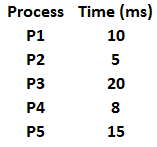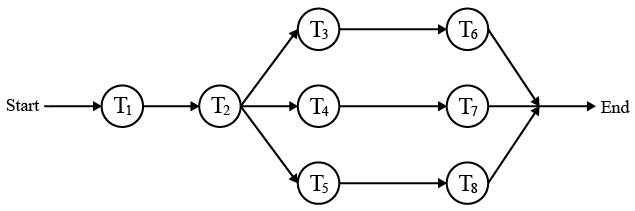# ISRO Scientist or Engineer Computer Science 2011

Instructions

For the following questions answer them individually

Question 1

# The encoding technique used to transmit the signal in giga ethernet technology over fiber optic medium isQuestion 2

# Which of the following is an unsupervised neural networkQuestion 3

# In compiler terminology, reduction in strength meansQuestion 4

# The following table shows the processes in the ready queue and time required for each process for completing its job.If round robin scheduling with 5 ms is used what is the average waiting time of the processes in the queue?Question 5

# MOV [BX], AL type of data addressingis calledQuestion 6

# Evaluate (X xor Y) xor YQuestion 7

# Which of the following is true about the z-buffer algorithm?Question 8

# What is the decimal value of the floating-point number C1D00000 (hexadecimal notation)? (Assume 32-bit, single precision floating point IEEE representation)Question 9

# Whatis the raw throughput of USB 2.0 technology?Question 10

# Below is the precedence graph for a set of tasks to be executed on a parallel processing system S.What is the efficiency of this precedence graph on S if each of the tasks $$T_{1},..., T_{8}$$ takes the same time and the system S has five processors?OR